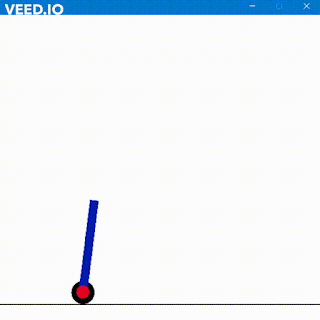## Basics of Neurons

Hey everyone, long time no see. I have been working on some stuff so was busy and away from my blog. Well, as for my interest in AI, I have been working on neural networks and its implementation which I'll discuss in this post.

In this post, you will learn:
• The basic concepts used in building a neural network
• Implementing logistic regression
• Single Neural Network
• Forward propagation and Backward propagation
• Building a small neural network using TensorFlow
• Building a deep neural network using TensorFlow.
As we know, we humans learn from our experiences and through training. Our brain runs a very high speed and processes any activity quickly. Well, it actually depends on the type of activity, the person is working on. But as we all know, humans have a high grasping power and easily learn, understand, classify, remember and do various other things which machines take a hell lot of time to compute. All these computations are processed by our mighty brain. So to understand the technical neural network, we must understand the biological neural network.

Source: Wikipedia

The brain consists of a huge number of neurons. These neurons are the fundamental unit of the brain. They receive electric signals as input from the external world (Hello World!!) which are processed and sent to other neurons. A basic neuron consists of axion, dendrites, action potential, synapse etc.

Axons are the thin structure and are called as the transmitting part of the neurons. Dendrite is the receiving part of the neuron which receives its input from synapse summing total inputs. The action potential helps the neurons to communicate with each other. So basically, neurons receive electrical impulses from dendrites, which informs the neuron about the outcome of an incident. If the outcome is not in favor, these impulses are altered by chemical and electrical reactions and sent to other neurons by neurotransmission. This keeps on happening until the brain achieves perfection.

In a similar way, we will work on an Artificial neuron.
Matrices and its manipulation is a basic requirement for this post. To simplify, we will work on the dot product, vectors, and broadcasting in python.

Dot Product
If A is a C × D matrix and B is a D × E matrix, then the dot product of A and B is a C × E matrix. The dot product will reduce our computation time whenever we have quite a lot of equations. Note that the number of columns in the first matrix should always be equal to the number of rows in the second matrix. In python, we will use dot() function from the NumPy package.

A typical example describing the dot() function in the NumPy package

import numpy as np
a = np.array([[7,6],[2,5]])
b = np.array([[10,6,70],[18,9, 11]])
c = np.dot(a,b)
print("Shape of A ", a.shape)
print("Shape of B ", b.shape)
print("Shape of C ", c.shape)
print("The dot product of A and B is : ", c)

And the result is

Broadcasting in Python helps to perform element-wise calculation between a matrix and vector or scalar. Scalar is the just a single number whereas a vector is rank 1 array. The advantage of NumPy package is that it provides all these operations on matrices, vectors, reshaping, transform etc.A vector can be represented as numpy.ndarray(n-Dimensional Array) object using NumPy's array() function. A column vector is of shape (A × 1) and a row vector is of shape (1 × B)

To shape an array we use the syntax Array_name.reshape(row, column)
To find the rank of the matrix we will use the function numpy.linalg.matrix_rank()

These were the basics required to start a simple program or project on neural networks. In the next post, I'll deal with Logic Regression with a single neuron which requires the basics as I have described above.

#### 1 comment:

1.### Self Balancing Robot using Machine Learning

Hola Amigos, I have always loved inverted pendulums. They are very fascinating to me and I play with them a lot. In real life, I have m...